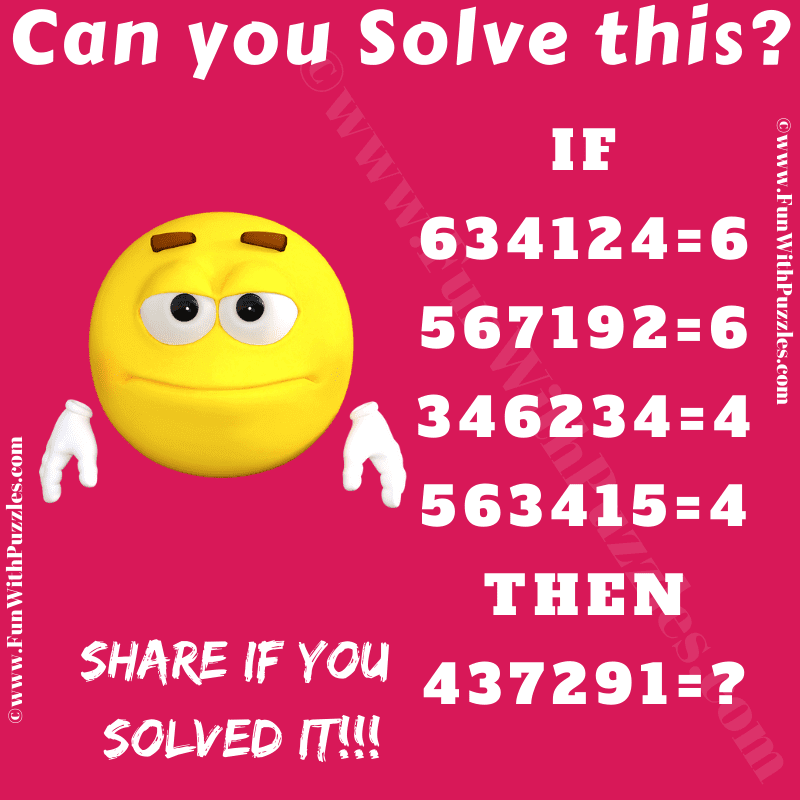This is a very interesting logic puzzle of mathematics for school students. In this maths logic puzzle, you are shown some number equations. These number equations are not mathematically true but these are the logical equations. Each logical number equation follows a certain hidden pattern or logical formula which makes these equations correct. Your challenge in this logic puzzle is to crack the logical code in these number equations. Once you are able to find the hidden logic, find the value of the missing number in the last equation.Can you solve this Maths Logic Puzzle?

The answer to this "Logic Puzzle of Mathematics", can be viewed by clicking on the answer button.